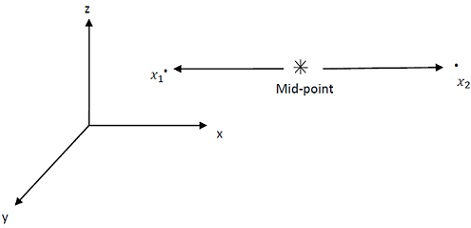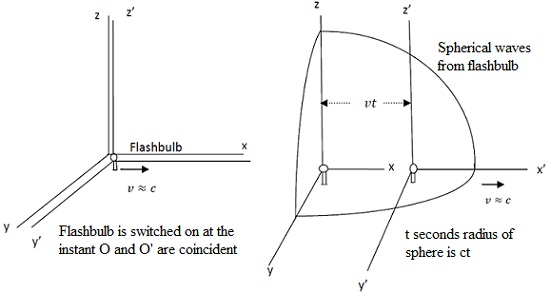#### Einstein Postulates and Lorentz Transformation, Physics tutorial

Einstein's Postulates:

Einstein's special theory of relativity comprise of two postulates that is,

I. Physical laws are same for all inertial frames of reference. As a result, all inertial frames are equal.

Postulate stresses that it is impossible by means of any physical measurement to find the state of absolute motion or universal frame of reference.

II. Velocity of light in free space has same value in all inertial frames of reference and is independent of motion of source.

These postulates, as you can see, seem to be anything but radical. Immediate consequence of first postulate was compelling need to alter Galilean transformation.

Second postulate on other hand has to do with finite velocity of interaction between particles. The interaction between two particles is explained in terms of their potential energy. That is to say, interaction is the function of position. Therefore, when one particle changes position (moves), other particle is influenced to move relative to it instantaneously. This signifies that signal of interaction propagates at infinite velocity. This supposition is inherent in Galilean transformation. Change in one body needs the finite time interval to start to manifest in another body at distance. Thus, signal of interaction propagates at finite velocity and this velocity is maximum. Clearly, there can't be any motion with velocity greater than this in nature. This is exactly what second postulate defines. In other words, velocity of propagation of signal of interaction-the velocity of light in free space-is same in all inertial frames of reference and is limiting velocity. No material object can be accelerated to the velocity in excess of c, velocity of light in free space.

Simultaneity:

Consider fourth of Galilean transformation equations, that is

t' = t

Equation appears to say that time is absolute or universal for all inertial frames. The essential procedure involved in measurement of time is measurement of simultaneity. Therefore, if we say that plane lands at 4 o'clock," what this means is that two events are simultaneous at same location namely, 1. The short hand of the clock is at 4 while long hand is at 12. There should be difference in time between two events at different locations and so they are no longer simultaneous. This is point where Galilean transformation is wrong by supposing that time of occurrence of two events at different locations is same. In other words, equation t' = t is not correct and should be altered.

The realistic method of determination of simultaneity needs use of real signal with highest known velocity that is electromagnetic wave like light. This leads us to Einstein's definition of simultaneity that defines that two instants of time t1 and t2, observed at two points x1 and x2 in particular frame of reference, are simultaneous if light signals simultaneously emitted from geometrically measured midpoint between x1 and x2 arrive at x1 at t1 and at x2 at t2. Converse is also true i.e.t1 and t2 are simultaneous if light signals emitted at t1 from x1 and t2 from x2 arrive at mid-point simultaneously. These definitions intimately mix times t1, t2 and spatial coordinates x1, x2. In Einstein's theory simultaneity doesn't have absolute meaning, independent of spatial coordinates, as it does in classical theory.Consequence of these definitions is that two events that are simultaneous in inertial frame of reference are in general not simultaneous in another inertial frame of reference in relative motion to it. Assume the box car is travelling to right at a very high constant velocity v. The high-speed flashbulb B is placed at geometrical centre of car where observer O' is located. Bulb is prepared with reflectors so that it can send light pulses in opposite directions when it is switched on. Photocells are fitted at opposite ends allow observer detect when light pulses strike ends of the car. At instant O' is abreast of O, observer at rest with respect to earth, flashbulb is switched on. Notice that two observers are in inertial reference frames (one is boxcar and other is earth), and so speed of light should be constant to both of them. To observer O' flashbulb is at rest relative to his reference frame. When bulb is switched on, light pulses travel equal distances in equal times to two ends C'1 and C'2 of car. For him experiment is same whether or not car is in motion. Notice that pulses of light will also travel equal distances to C1 and C2 he ends of boxcar as estimated by O in equal times if two observers are abreast and O' is at rest relative to O. So, light pulses hit two ends of car simultaneously.

Now, to stationary observer o, C'1 approaches while C'2 recedes from him. Therefore, for him, distance traveled by light pulse to left end of car OC'1 is shorter than distance to right end of car OC'2. Light pulses to C'1 arrive before those traveling to C'2. Notice that light pulses from O' arrive C2 ahead of light pulses from O' to C1.

Lorentz Transformation:

Consider two observers O and O' in two inertial frames of reference S and S', who view same event, with S' moving in x-direction relative to S at constant velocity V as in figure. Relative to respective coordinate systems O allots location and time coordinates of event as (x, y, z, t) while O' assigns (x', y', z', t') as location and time coordinates for same events. Desired coordinate transformation comprises of the set of algebraic equations that connect two set of coordinates, i.e., relation which permits us to compute primed set of coordinates in terms of unprimed ones and vice versa. Galilean relativity gave these relations as in equation, i.e., y' = y, z' = z, t' = t and x' = x - vt. Of course, this conforms with common experience provided v << c, that is, relative motion of coordinate systems takes place at ordinary velocity. Though, at relativistic velocity, i.e., at velocity close to that of light (v ≈ c), these transformation relations oppose Einstein's postulates.Let us suppose that at instant origins of two frames are coincident, flash bulb explodes as shown in figure. The light sphere then expands in all directions at velocity c. At the instant after bulb had exploded, observer O in S frame observes that moving frame has travelled the distance x = vt in -direction as shown in figure, and that radius of expanding light sphere is r = ct. You will recollect, from study of coordinate geometry,

That r2 = x2 + y2 + z2.

Thus, x2 + y2 + z2 = c2t2

Observer O' in frame observes light sphere with radius r' = ct'.

Thus, x'2 + y'2 + z'2 = c2t'2

Now, from Galilean transformation, we have y' = y, z' = z, t' = t and x' = x - vt

Solving these equations we get:

x2 - 2xvt + v2t2 + y2 + z2 = ct

By solving equations we obtain

x' = γ(x - vt) = (x- vt)/(√(1 - β2))

y' = y

z' = z

and t' = β(t - βx/c)

= (t - vx/c2)/(√(1 - β2))

The set of equations are known as the Lorentz- Einstein relations or so-called Lorentz transformations.

Inverse Lorentz transformation equations are obtained simply by replacing v by -v in corresponding transformation equations. After doing this we obtain following set of

x = γ(x' - vt') = (x' - vt')/(√(1 - β2))

y = y'

z = z

and t = γ(t' - βx'/c)

= (t' - vx'/c2)/(√(1 - β2))

These equations allow us to transform from primed frame to unprimed frame.

Properties of the Lorentz Transformation:

Relativity of Simultaneity:

The most salient characteristic of Lorentz - Einstein relations is transformation of time that shows relativity of simultaneity. Consider two frames inertial frames of reference S and S' that are in relative motion in common positive x - direction with constant velocity. Consider two events, like explosion of a pair of flash bulbs which happen concurrently at points B and C in S' the frame. For observer at mid-point A of distance between B and C in this frame, the times tB and tC of occurrence of said events at B and C are equal i.e. t'B = t'C or time interval between the events t'B - t'C = 0.

Though, for observer in the S frame, the times for the events tB and tC are related to those in S' frame by Lorentz equations.

Therefore, tB = γ(t'B + β(x'B/C))

and

tC = γ(t'C + β(x'C/C))

Time interval between two events for this observer is:

tB - tC = γ{t'B - t'C + β/C(x'B - x'C)} ≠ 0

Symmetry of Relations:

Carefully, examine equations. You will realize that they are symmetric not only in x but also in ct. Let us try to verify assertion. Simply replace t in equation by τ/c and t' by τ'/c and then divide second equation by c, that is,

x' = γ(x - v(τ/c)) and τ'/c = γ(τ/c - β(x/c))

Thus

β = γ(τ - βx) or τ' = γ(τ - vx/c)

By comparison, It is easily seen that equations are symmetric if τ = ct and τ' = ct'.

Importance of Lorentz factor γ:

For ≠ 0, Lorentz factor γ is always greater than unity. Besides, as v → c, γ → ∞. Also, v > c leads to the imaginary value of γ and for v<< c, Lorentz transformation reduces to Galilean transformation. Physical meaning of all of this is that relative velocity of two inertial frames of reference should be less than c, as finite real coordinates in one frame should correspond to finite real coordinates in any other frame.

Relationship between Coordinates and Differentials:

As standard Lorentz transformation is linear and homogeneous, coordinate differences and differentials satisfy same transformation equations as coordinates themselves. In other words,

Δx' = γ(Δx - vΔt)

Δy' = Δy

Δz' = Δz

Δt' γ(Δt - β(Δx/c)) and

dx' = γ(dx - vdt)

dy' = dy

dz' = dz

Δt' = γ(dt - β(dx/c))

Invariance of Maxwell's Equation:

Among successes of Lorentz -Einstein transformation, it should be able to show invariance of both mechanical and electromagnetic laws of physics. Any transformation which should replace Galilean transformation should satisfy requirement. We now proceed to show invariance of Maxwell's equations in light of special relativity and Lorentz -Einstein transformation.

Electromagnetic wave equation that is derived from Maxwell's equations is:

2Ex/∂x2 + ∂2Ex/∂y2 + ∂2Ex/∂z2 = (1/c2)(∂2Ex/∂t2)

Now use chain rule to write first derivative of electric field component of wave equation and substituting the values in wave equation, we get

2Ex/∂x2 + ∂2Ex/∂y2 + ∂2Ex/∂z2 - (1/c2)(∂2Ex/∂t2) = ∂2Ex/∂x'2 + ∂2Ex/∂y'2 + ∂2Ex/∂z'2 - (1/c2)(∂2Ex/∂t'2)

Thus, the equation of electromagnetic waves and consequently Maxwell's equations of electromagnetic phenomena are invariant (retain same form) under Lorentz transformation.

Tutorsglobe: A way to secure high grade in your curriculum (Online Tutoring)

Expand your confidence, grow study skills and improve your grades.

Since 2009, Tutorsglobe has proactively helped millions of students to get better grades in school, college or university and score well in competitive tests with live, one-on-one online tutoring.

Using an advanced developed tutoring system providing little or no wait time, the students are connected on-demand with a tutor at www.tutorsglobe.com. Students work one-on-one, in real-time with a tutor, communicating and studying using a virtual whiteboard technology.  Scientific and mathematical notation, symbols, geometric figures, graphing and freehand drawing can be rendered quickly and easily in the advanced whiteboard.

Free to know our price and packages for online physics tutoring. Chat with us or submit request at info@tutorsglobe.com